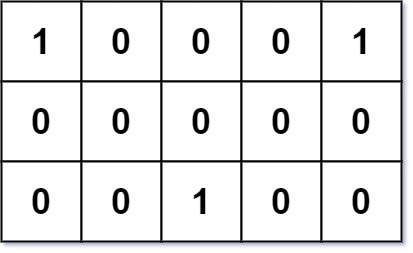### LeetCode• ㊗️
• 大家
• offer
• 多多！

## Problem

Given an m x n binary grid grid where each 1 marks the home of one friend, return the minimal total travel distance.

The total travel distance is the sum of the distances between the houses of the friends and the meeting point.

 The distance is calculated using Manhattan Distance, where distance(p1, p2) = p2.x - p1.x + p2.y - p1.y .

Example 1:Input: grid = [[1,0,0,0,1],[0,0,0,0,0],[0,0,1,0,0]]
Output: 6
Explanation: Given three friends living at (0,0), (0,4), and (2,2).
The point (0,2) is an ideal meeting point, as the total travel distance of 2 + 2 + 2 = 6 is minimal.
So return 6.


Example 2:

Input: grid = [[1,1]]
Output: 1


## Code

class Solution {
public int minTotalDistance(int[][] grid) {
int m = grid.length;
int n = grid.length;

List<Integer> l1 = new ArrayList<>();
List<Integer> l2 = new ArrayList<>();

for(int i = 0; i < m; i++){
for(int j = 0; j < n; j++){
if(grid[i][j] == 1){
}
}
}

Collections.sort(l1);
Collections.sort(l2);

int left = 0;
int right = l1.size() - 1;

int res = 0;
while(left < right){
res += l1.get(right) - l1.get(left);
res += l2.get(right) - l2.get(left);
left++;
right--;
}

return res;
}
}